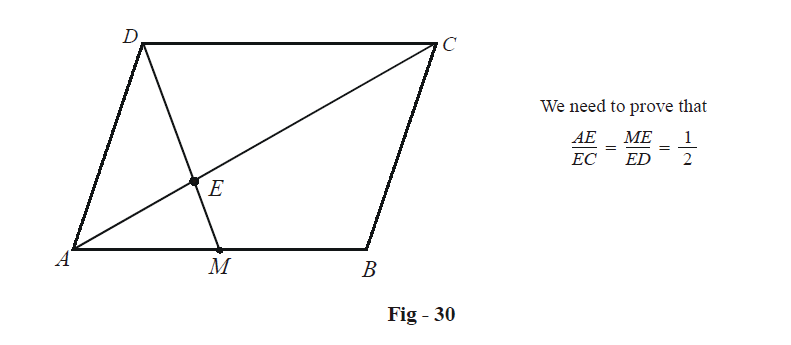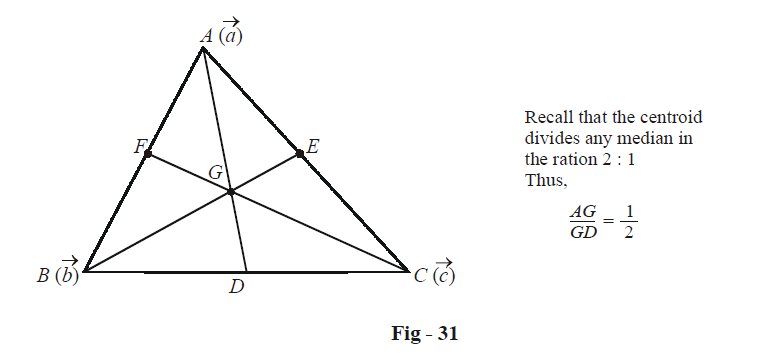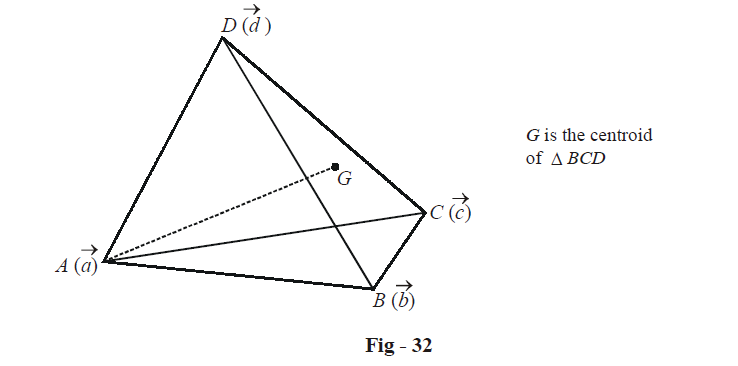# Examples On Basics Of Vectors Set-3

Go back to  'Vectors and 3-D Geometry'

Example – 11

In a parallelogram ABCD, let M  be the mid-point of AB. AC and MD meet in E. Prove that both AC and MD are trisected at E.

Solution:There’s no loss of generality in assuming A to be the origin $$(\vec 0)$$ . Let B and C have the position vectors $$\vec b\,\,{\text{and}}\,\,\vec c$$. The position vector of D is given by $$\overrightarrow {AD}$$ where

\begin{align}&\overrightarrow {AD} = \overrightarrow {AC} + \overrightarrow {CD} \hfill \\\\&\;\quad= \vec c - \overrightarrow {AB} \hfill \\\\&\quad\;= \overrightarrow c - \overrightarrow b \hfill \\ \end{align}

D is therefore the point  $$\overrightarrow c - \overrightarrow b$$.

Since M is the mid-point of AB, M's position vector is $$\frac{{\vec b}}{2}$$. Let us first find the position vector of a point E' which lies on AC and trisects it. We’ll then show that same point lies on DM and trisects on DM and trisects it too, proving the stated assertion.

$E' \equiv \frac{{1 \times \vec c + 2 \times \vec 0}}{3} = \frac{{\vec c}}{3}$

A point E'' which trisects MD is

$E' \equiv \frac{{2 \times \frac{{\vec b}}{2} + 1 \times \left( {\vec c - \vec b} \right)}}{3} = \frac{{\vec c}}{3}$

Since E' and E'' are the same-point, say E, we see that AC and MD are trisected at E.

Example – 12

Prove that the lines joining the vertices of a tetrahedron to the centroids of the opposite faces are concurrent.

Solution: It is in this example that the powerful nature of vector algebra will become apparent; this is a 3-D problem and any other methods of proving the assertion will be extremely cumbersome. For those of you who are more mathematically inclined, you can try proving the assertion using already known methods.

First of all, we need to know how to write the position vector of the centroid of a triangle in terms of the position vectors of its vertices.Since D is the mid-point of BC, we have

$D \equiv \frac{{\vec b + \vec c}}{2}$

Since AG : GD = 2 : 1, we can now determine G:

$G \equiv \frac{{2 \times \left( {\frac{{\vec b + \vec c}}{2}} \right) + 1 \times \vec a}}{{2 + 1}} = \frac{{\vec a + \vec b + \vec c}}{3}$

We now consider a tetrahedron, say, with the vertices $$A\left( {\vec a} \right),\,\,B(\vec b),\,\,C\,\left( {\vec c} \right){\text{and}}\,\,D\,(\vec d)$$ .Consider the centroid of $$\Delta BCD$$ , say G, which will be given by

$G \equiv \frac{{\vec b + \vec c + \vec d}}{3}$

Now, pause and think about the assertion we are required to prove. If the four lines are indeed concurrent, the point of concurrency must be given by a position vector which has a ‘symmetrical’ expression with respect to all the four vertices. Can you find a point on the segment AG which has a symmetrical expression with respect to the four vertices?

A little thought will show that the answer is yes: consider the point P which divides AG in the ratio 3: 1. P will be given by

$P \equiv \frac{{3 \times \left( {\frac{{\vec b + \vec c + \vec d}}{3}} \right) + 1 \times \vec a}}{{3 + 1}} = \frac{{\vec a + \vec b + \vec c + \vec d}}{4}$

It is immediately apparent now that P lies on each of the four lines joining the vertices to centroids of the opposite faces! In addition, we’ve also been able to find the position vector of P in terms of the position vectors of the four vertices of the tetrahedron.

Vectors
grade 11 | Questions Set 1
Vectors